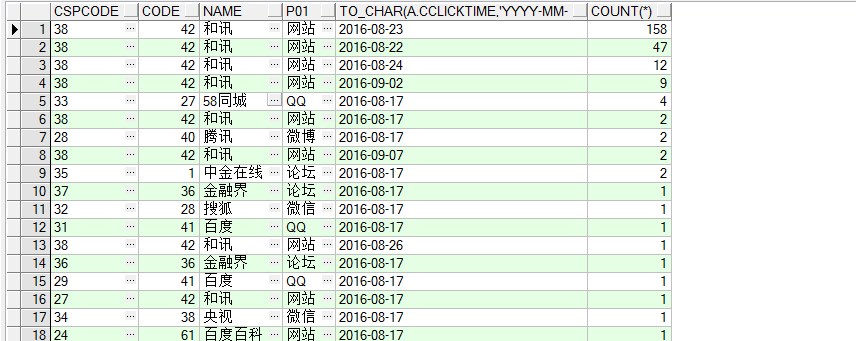【急】[在线等]PLSQL 查询前十条 伪列加上排名

select distinct(a.cspcode), b.ccode as code ,t_c.cname as name,t_d.cname as p01,to_char(a.cclicktime,'yyyy-mm-dd'),count(*) from t_spead_clickqty a
inner join t_page_channels b on a.cspcode=b.cspcode
inner join t_channels t_c on t_c.ccode = b.ccode
inner join t_dictionary t_d on t_c.cchanneltype = t_d.ccode
where 1=1 and a.cclicktime>=to_date('2016-08-10','yyyy-mm-dd') and to_date('2016-09-10','yyyy-mm-dd')>=a.cclicktime

group by a.cspcode,t_c.cname,b.ccode,t_d.cname,to_char(a.cclicktime,'yyyy-mm-dd')
order by count(*) desc4个回答

select * from (
select distinct(a.cspcode) as id, b.ccode as code ,t_c.cname as name,t_d.cname as p01,to_char(a.cclicktime,'yyyy-mm-dd') as p02,count(*) as p03 from t_spead_clickqty a
inner join t_page_channels b on a.cspcode=b.cspcode
inner join t_channels t_c on t_c.ccode = b.ccode
inner join t_dictionary t_d on t_c.cchanneltype = t_d.ccode
where 1=1 and a.cclicktime>=to_date('2016-08-10','yyyy-mm-dd') and to_date('2016-09-10','yyyy-mm-dd')>=a.cclicktime

group by a.cspcode,t_c.cname,b.ccode,t_d.cname,to_char(a.cclicktime,'yyyy-mm-dd')
order by count(*) desc
) where rownum<=10

select * from table where rownum >=10sinat_25186077 你这个确定能 查出来数据?

select * from table order by rownum limit 10-

-